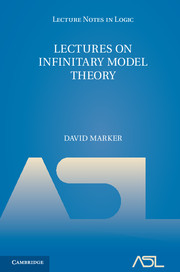# Lecture Notes in Logic, 46

## Lectures on Infinitary Model Theory

David MarkerYear: 2016

ISBN-13: 9781316859605
183 pages. Hardcover.
Buy now (when ordering, include the discount code ASL2016 to receive the 25% ASL member discount)

Infinitary logic, the logic of languages with infinitely long conjunctions, plays an important role in model theory, recursion theory and descriptive set theory. This book is the first modern introduction to the subject in forty years, and will bring students and researchers in all areas of mathematical logic up to the threshold of modern research. The classical topics of back-and-forth systems, model existence techniques, indiscernibles and end extensions are covered before more modern topics are surveyed. Zilber’s categoricity theorem for quasiminimal excellent classes is proved and an application is given to covers of multiplicative groups. Infinitary methods are also used to study uncountable models of counterexamples to Vaught’s conjecture, and effective aspects of infinitary model theory are reviewed, including an introduction to Montalbán’s recent work on spectra of Vaught counterexamples. Self-contained introductions to effective descriptive set theory and hyperarithmetic theory are provided, as is an appendix on admissible model theory.

• Introduction

Part I. Classical Results in Infinitary Model Theory:

1. Infinitary languages
2. Back and forth
3. The space of countable models
4. The model existence theorem
5. Hanf numbers and indiscernibles

Part II. Building Uncountable Models:

1. Elementary chains
2. Vaught counterexamples
3. Quasinimal excellence

Part III. Effective Considerations:

1. Effective descriptive set theory
2. Hyperarithmetic sets
3. Effective aspects of Lω1,ω
Spectra of Vaught counterexamples
• Appendix A. N1-free abelian groups; Appendix B. Admissibility; References
• Index